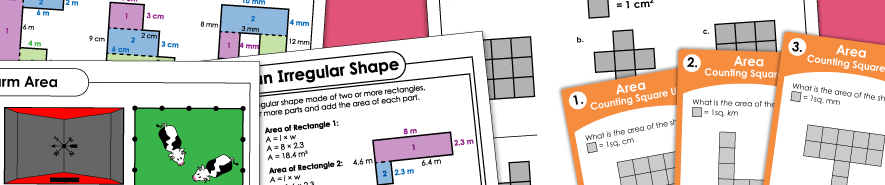# Area Worksheets## Rectangles and Rectilinear Figures

Area: Counting Square Units (Very Basic)

Find the area of the shapes by counting the number of square unit tiles shown. These are very basic-level worksheets.

Areas of Rectangles and Squares

Find the areas of the rectangles and squares by using the formula area = length times width.

Areas of Irregular Shapes (Rectilinear Figures)

These worksheets have irregular shapes (made of 2 or more rectangles; rectilinear figures). Students find the areas of the individual rectangles and add them together.

## Areas of Other Shapes

Areas of Circles

These PDFs have circles with the radius or diameter shown. Students must calculate the areas of the circles using the correct formula.

Areas of Parallelograms

Here you'll find a series of worksheets on area of parallelograms.

Areas of Trapezoids

Practice finding the area of trapezoids with these task cards and worksheets.

Areas of Triangles

This page has a collection of worksheets for calculating the areas of triangles.

## Surface Area

Surface Area

Calculate the surface area of rectangular prisms, pyramids, cylinders, spheres, and irregular solid shapes.

Perimeter

Find the perimeter of various polygons with these printable games, activities, and worksheets.